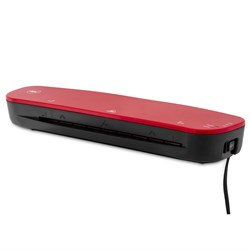Select Office Supplies

# Laminating Machines

\$140.90 \$155.00 inc GST ex GST (EACH)
Increase value Decrease value
\$340.90 \$375.00 inc GST ex GST (EACH)
Increase value Decrease value
\$54.54 \$60.00 inc GST ex GST (EACH)
Increase value Decrease value
\$72.71 \$79.99 inc GST ex GST (EACH)
Increase value Decrease value
\$599.09 \$659.00 inc GST ex GST (EACH)
Increase value Decrease value
\$799.09 \$879.00 inc GST ex GST (EACH)
Increase value Decrease value
\$142.72 \$157.00 inc GST ex GST (EACH)
Increase value Decrease value
\$154.54 \$170.00 inc GST ex GST (EACH)
Increase value Decrease value
\$499.09 \$549.00 inc GST ex GST (EACH)
Increase value Decrease value
\$1271.82 \$1399.00 inc GST ex GST (EACH)
Increase value Decrease value
\$244.54 \$269.00 inc GST ex GST (EACH)
Increase value Decrease value
\$253.63 \$279.00 inc GST ex GST (EACH)
Increase value Decrease value
\$326.36 \$359.00 inc GST ex GST (EACH)
Increase value Decrease value
\$144.54 \$159.00 inc GST ex GST (EACH)
Increase value Decrease value
\$451.81 \$497.00 inc GST ex GST (EACH)
Increase value Decrease value
\$262.72 \$289.00 inc GST ex GST (EACH)
Increase value Decrease value
\$264.54 \$291.00 inc GST ex GST (EACH)
Increase value Decrease value
\$109.08 \$119.99 inc GST ex GST (EACH)
Increase value Decrease value
\$139.08 \$152.99 inc GST ex GST (EACH)
Increase value Decrease value\$17.27 \$19.00 inc GST ex GST (EACH)
Increase value Decrease value
##### Products to compare:
Comparing Products